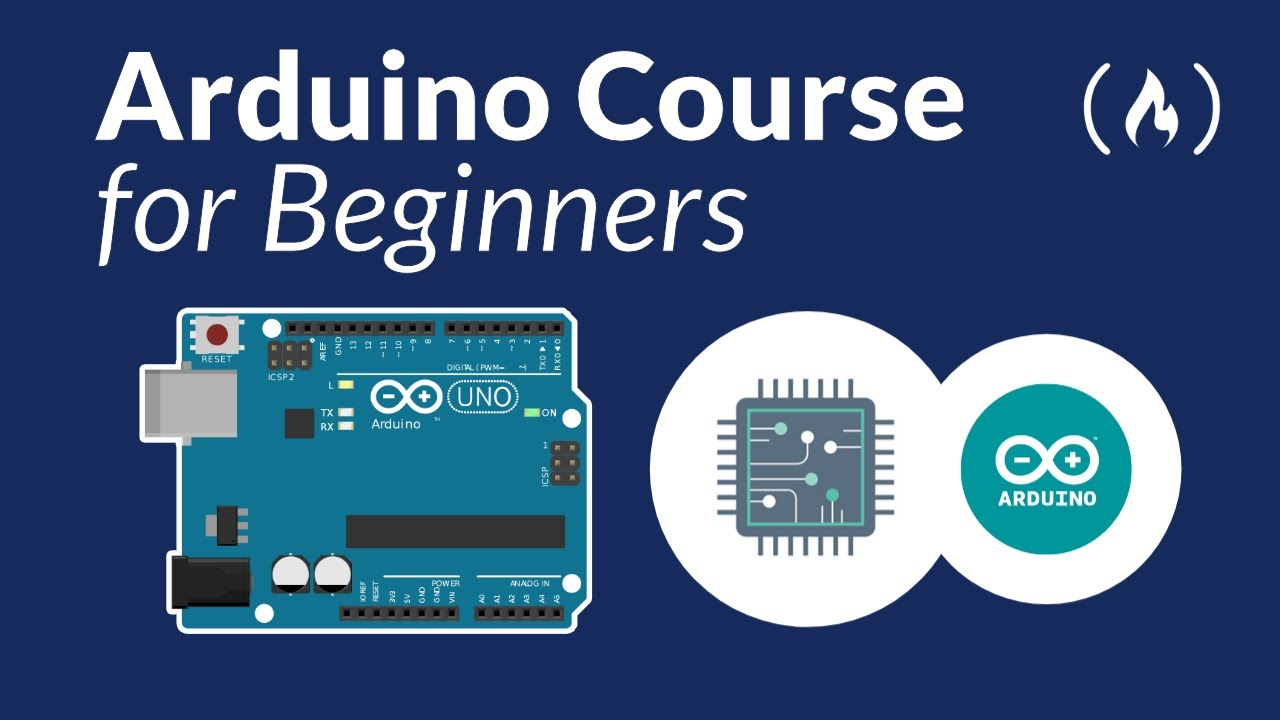# Arduino Course for Beginners - Open-Source Electronics PlatformLearn how to use Arduino hardware and software in this full course for beginners. Arduino is an easy-to-use, open-source electronics platform Arduino boards are able to read inputs - light on a sensor, a finger on a button, or a Twitter message

Learn how to use Arduino hardware and software in this full course for beginners. Arduino is an easy-to-use, open-source electronics platform Arduino boards are able to read inputs - light on a sensor, a finger on a button, or a Twitter message - and turn it into an output - activating a motor, turning on an LED, publishing something online. You can tell your board what to do by sending a set of instructions to the microcontroller on the board.

No hardware is required for to follow along with this course!

✏️ Course developed by Ashish Bansal.

⭐️Course Contents ⭐️
Section 1: Objective of the course
(0:00) Course Introduction

(01:21) Section 2: Foundation of Electronics
(01:36) Electricity
(02:10) Static Electricity
(03:37) Current Electricity
(04:12) Voltage
(06:09) Current
(08:45) Resistance
(10:05) Ohm’s Law
(11:55) Ohm’s Law Example
(13:46) Resistances in Series and Parallel
(26:03) Resistance Color Coding

(28:26) Section 3: Intro to Arduino Board
(28:46) What is Microcontroller and Microprocessor
(31:16) What category Arduino falls into?
(31:33) Different Types of Arduino Boards
(33:04) Parts of Arduino Uno
(35:52) Technical Specifications of Arduino Uno

Section 4: Intro to Arduino IDE
(38:58) What is IDE?
(43:08) Testing the Arduino.
(44:22) What if you don’t have an Arduino board?

(46:34) Section 5: Before we move ahead
(49:16) How to make connections in breadboard?
(1:00:10) Some safety instructions and Do’s and Don’ts
(1:01:53) Input & Output
(1:08:47) Analog & Digital
(1:14:04) Bit & Byte

(1:16:26) Section 6: Arduino Programming
(1:16:46) Introduction
(1:17:41) The First Step into Programming
(1:19:37) Bare minimum structure of an Arduino Program
(1:21:37) White Spaces and Case Sensitivity
(1:24:06) pinMode
(1:26:44) digitalWrite and delay
(1:29:51) Camel casing

Section 6.1 Introduction to Variables and Data Types
(1:30:51) What are variables and data types
(1:31:31) Int data type
(1:35:11) Arithmetic operators
(1:41:51) Incrementing and Decrementing our variables
(1:44:14) Float data type
(1:46:48) Bool/Boolean data type
(1:49:24) Byte data type
(1:50:27) Char data type
(1:52:46) Conclusion

Section 6.2 Variable Scope and Qualifiers
(1:53:19) What is Scope? Global and Local Variables
(1:57:59) What are Qualifiers, starting with const qualifier
(1:59:51) Alternative to const qualifier: #define
(2:01:55) Static Qualifier

Section 6.2 Comparison and Logical Operators
(2:04:25) What are comparison operators?
(2:08:58) What are Logical Operators?

(2:13:16) Section 6.3 Control Structures
(2:14:21) if statement
(2:20:47) else statement
(2:24:24) A joke :P
(2:25:10) if - else Simulation
(2:29:27) Introduction to loop control structures
(2:30:52) For loop
(2:41:02) While loop
(2:45:49) do…while loop
(2:50:16) break
(2:52:24) continue
(2:55:05) return
(2:56:41) switch…case

Section 6.4 Remaining data types
(3:01:30) Arrays
(3:09:34) Strings

Section 6.5 Functions
(3:15:14) What are functions?